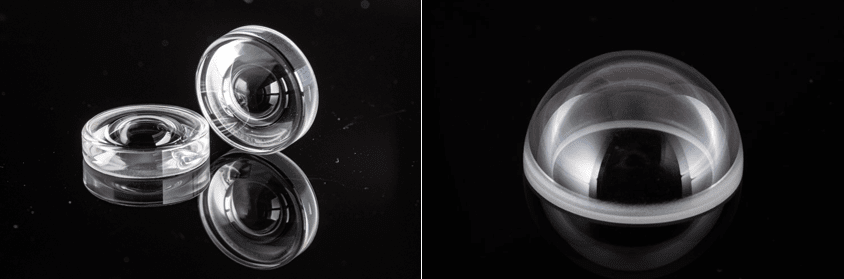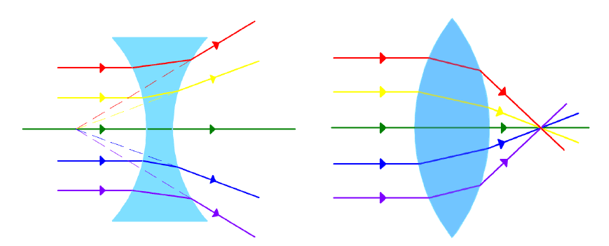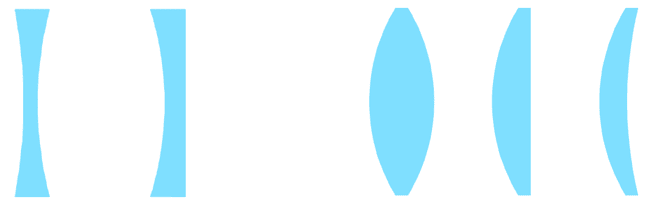## Lesson 5 – Intro to Optical Components

Posted on: Friday, February 18th, 2022  In: Learning Optics with Austin

The properties of light evident in nature are utilized by humans through instruments such as lenses and mirrors. While one might think that glass is the main material used to make lenses, resin has become the most common material due to its lightness yet hardness (resistance to scratching). Lenses refract light while mirrors reflect light.Glass slab

Other than being made of glass, traditional lenses tend to be spherical lenses which are characterized by having a bump or curve. Thinner aspherical lenses produce a better image than spherical lenses and have thus supplanted spherical lenses as the better choice.Aspherical (left) vs. Spherical (right) lenses

Another common way of categorizing lenses is convex versus concave. A convex lens has a thicker center compared with its edge, while a concave lens has a thicker edge than its center. Since light converges after passing through convex lenses, these lenses are also called converging lenses. As concave lenses have the opposite effect, they are known as diverging lenses.Concave (left) vs. Convex (right)

There is however, another pair of names synonymous to convex and concave- positive and negative respectively. The positive and negative labels have to do with focal length. In short, we know that light converges after passing through a convex lens. The point at which light converges is called the focal point and the length from the lens to that point is called the focal length. Convex lenses have a positive focal length because the focal point exists. For concave lenses however, light diverges after passing through. Instead of finding the length to a focal point, the diverging light is traced backwards to a single point prior to entering the lens. This point is called the virtual focal point and the distance to it is the negative focal length.Group of Concave (left) vs. Convex (right) lenses

The introduction to lenses is the first step in understanding how the concepts of optics relates to the products we make. Stay tuned for the next post as we look at the different applications of focal length!

Next: Lesson 6 – Focal Length and Applications of Lenses